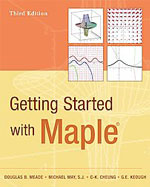Getting Started with Maple, 3rd ed. - Maplesoft Books - Maple Books, Maple Resources and Math BooksHome : Maplesoft Books : Getting Started with Maple, 3rd ed.Details Search# Getting Started with Maple, 3rd ed.

## 2009

Description

From the Preface:

Using this Guide

The purpose of this guide is to give a quick introduction on how to use Maple. It primarily covers Maple 12, although most of the guide will work with earlier versions of Maple. Also, throughout this guide, we will be suggesting tips and diagnosing common problems that users are likely to encounter. This should make the learning process smoother.

This guide is designed as a self-study tutorial to learn Maple. Our emphasis is on getting you quickly "up to speed." This guide can also be used as a supplement (or reference) for students taking a mathematics (or science) course that requirse use of Maple, such as Calculus, Multivariate Calculus, Advanced Calculus, Linear Algebra, Discrete Mathematics, Modeling, or Statistics.

Organization of the Guide

The Guide is organized as follows:

• Chapter 1 gives a short demonstration of what you'll see in the remaining parts of the Guide. Chapters 2 through 11 contain the basic information that almost every user of Maple should know.
• Chapters 12 through 15 demonstrate Maple's capabilities for single-variable calculus. This includes working with derivatives, integrals, series and differential equations.
• Chapters 16 through 21 cover topics of multivariate calculus. Here you'll find the discussion focusing on partial derivatives, multiple integrals, vectors, vector fields, and line and surface integrals.
• Chapters 22 and 23 introduce the statistical capabilities of Maple.
• Chaptesr 24 through 27 address a collection of topics ranging from animation and simulation to programming, and list processing.
• Two appendices explain how to learn more about Calculus and a quick reference guide.

Chapter Structure

Each chapter of the Guide has been structured around an area of undergraduate mathematics. Each moves quickly to define relevant commands, address their syntax, and provide basic examples.

Every chapter ends with as many as three special sections that can be passed over during your first reading. However, these sections will provide valuable support when you start asking questions and looking for more detail. These three sections are:

• More Examples
Here, you'll find more technical examples or items that address more mathematical points.
• Useful Tips
This section contains some simple pointers that all Maple users eventually learn. We've drawn them from our experiences in teaching undergraduates how to use Maple.
• Troubleshooting Q&A
Here we  present a question and answer dialogue on common problems and error messages. You'll also be able to find out how certain commands work or what they assume you know in using them. Many useful topics are covered here.
Categories:
Other Details
Language: English
ISBN: 978-0-470-45554-8
Publisher: Wiley
 Do you know of a book that should be included in our list? Tell us about it!
 Title/Author: Category: Any Category Abstract Algebra Biology Calculus Chemistry Complex Analysis Computer Algebra Computer Science Conference Proceedings Courseware Cryptography Differential Equations Differential Geometry Dynamical Systems Economics Engineering Games Linear Algebra Maple Manuals Mathematical Modeling Number Theory Numerical Analysis Operations Research Optimization Physics Pre-Calculus Probability & Statistics Programming Recent Releases Vector Calculus Language: Any Language English French German Spanish Japanese Dutch Russian Swedish Polish Persian ChineseAbstract Algebra Biology Calculus Chemistry Complex Analysis Computer Algebra Computer Science Conference Proceedings Courseware Cryptography Differential Equations Differential Geometry Dynamical Systems Economics Engineering Games Linear Algebra Maple Manuals Mathematical Modeling Number Theory Numerical Analysis Operations Research Optimization Physics Pre-Calculus Probability & Statistics Programming Recent Releases Vector Calculus